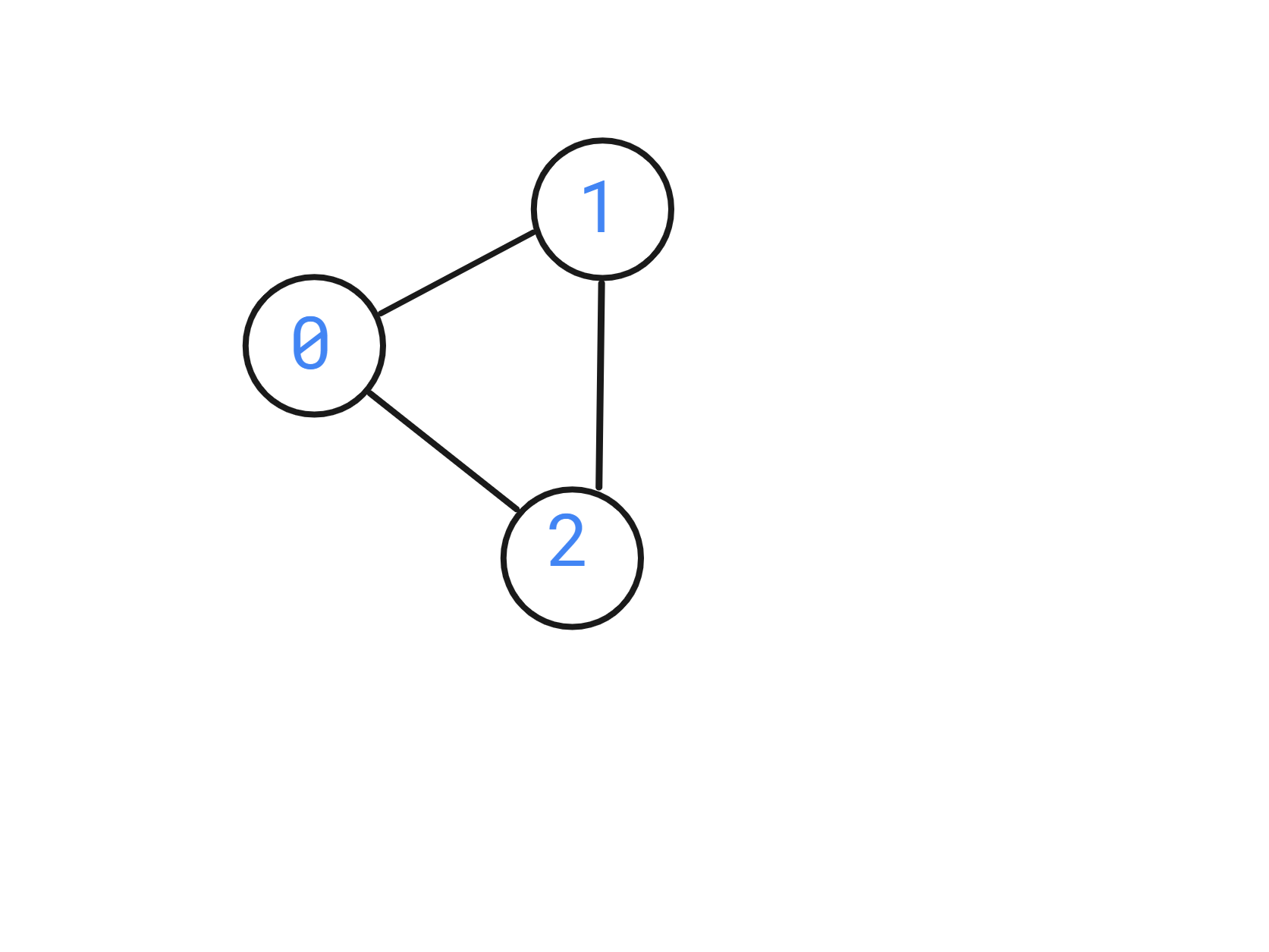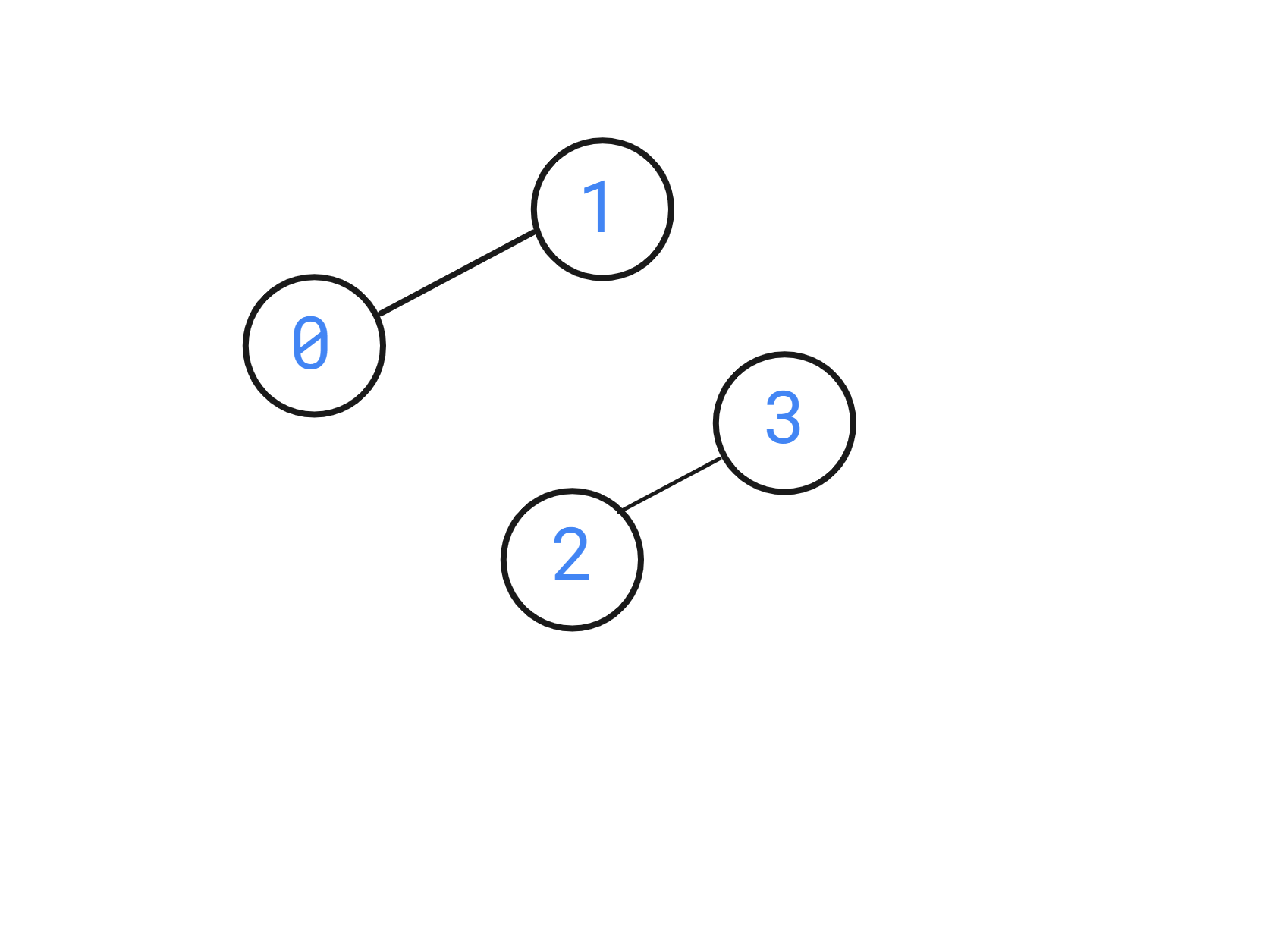New update is available. Click here to update.Close
Topic list
Check Bipartite Graph
MEDIUM
50 mins43 upvotes
Graph
Topics (Covered in this problem)
Problem solved
Skill meterGraph
-
-
Other topics
Problem solved
Skill meterStrings
-
-Matrices (2D Arrays)
-
--
-Sorting
-
-Binary Search
-
-Stacks & Queues
-
-Trees
-
-Dynamic Programming
-
-Greedy
-
-Tries
-
-Arrays
-
-SQL
-
-Binary Search Trees
-
-Heap
-
-Bit Manipulation
-
-
Solve problems & track your progress
Checkout your overall progress in every topic here
BecomeSensei
in DSA topics
Open the topic and solve more problems associated with it to improve your skills
Check out the skill meter for every topic
See how many problems you are left with to solve for cracking any stage. Score more than zero to get your progress counted.

# Check Bipartite Graph

Medium0/80
Avg time to solve 50 mins
Success Rate 50 %Share43 upvotes

## Problem Statement

#### You are given a 2D array ‘edges’ which contains 0 and 1, where ‘edges[i][j]’ = 1 denotes a bi-directional edge from ‘i’ to ‘j’.

##### Note:
``````If edges[i][j] = 1, that implies there is a bi-directional edge between ‘i’ and ‘j’, that means there exists both edges from ‘i’ to ‘j’ and to ‘j’ to ‘i’.
``````

#### For example

``````Given:
‘N’ = 3
‘edges’ = [[0, 1, 1], [0, 0, 1], [0,0,0]].
``````Detailed explanation ( Input/output format, Notes, Constraints, Images )#### Sample Input 1 :

``````2
4
0 1 0 0
0 0 0 1
0 0 0 0
0 0 0 0
3
0 1 1
0 0 1
0 0 0
``````

#### Sample Output 1 :

``````1
0
``````

#### Explanation of the Sample Input 1:

``````In the first test case, the graph is visualized as below,
````````````The graph can be divided into 2 disjointed sections, i.e. S1 = {0,2} and S2 = {1,3}. Therefore the answer is true.

In the second test case, the graph is visualized as below:
````````````The answer is 0 since there is no way this graph can be divided into 2 disjoint sets of points.
``````

#### Sample Input 2 :

``````2
4
0 0 1 0
0 0 0 1
0 0 0 0
0 0 0 0
3
0 1 1
0 0 0
0 0 0
``````

#### Sample Output 2 :

``````1
1
``````AutoConsole